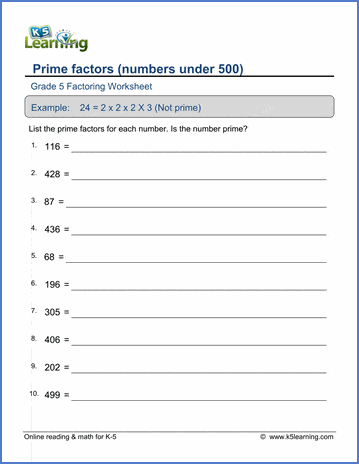## Factoring, GCF and LCM worksheets

Our grade 5 factoring worksheets provide students practice in factoring and finding prime factors of numbers and finding the greatest common factor and the the least common multiple of pairs of numbers.

## Factoring

List all the factors of the given number [0-100]
Prime factor trees
Prime numbers

## GCF and LCM

Find the greatest common factor (GCF) of 2 numbers between 1 and 100
Find the lowest common multiple (LCM) of 2 numbers between 2 and 30

## Word problems

GCF / LCM word problems